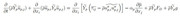## Equation of particle velocity

Questions and remarks about code_saturne usage
Forum rules
napken
Posts: 6
Joined: Wed Oct 01, 2014 12:30 pm

### Equation of particle velocity

Hello,

I'm using the heavy fuel combustion (icfuel = 0) in CS 5.0.4 and also activating the drift of the particle class (i_com_drift = 1). I'd like to know the expression of the transport equation of particle velocity solved in the code. Does the equation look like below?